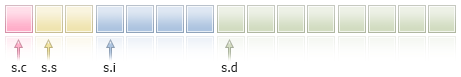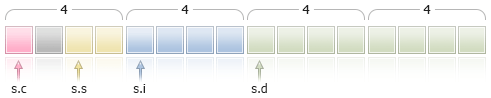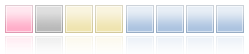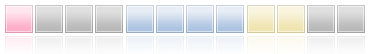# 架构和类 以及界面

## 架构

### 架构说明

 struct structure_name   {    elements_description   };

 #pragma pack(1)

 struct trade_settings   {    uchar  slippage;     // 许可的下降值-1字节大小    char   reserved1;    // 跳过 1 字节    short  reserved2;    // 跳过 2 字节    int    reserved4;    // 跳过另4个字节。确保定位8字节界限    double take;         // 固定利润价格值    double stop;         // 受保护的止损价格值   };

### 简单构造

• 如果对象属于相同的构造类型
• 如果对象通过血统连接，这意味着一个构造是另一个构造的后代。

 //--- 禁止复制不同类型的简单构造       my_tick1=last_tick;               // 这里编译器返回错误             //--- 同时也禁止不同类型构造彼此的类型转换       my_tick1=(CustomMqlTick)last_tick;// 这里编译器返回错误

 CustomMqlTick my_tick1,my_tick2;       //--- 允许复制CustomMqlTick以下相同类型的对象       my_tick2=my_tick1;             //--- 从CustomMqlTick简单构造对象创建一个数组并为其写值       CustomMqlTick arr;       arr=my_tick1;       arr=my_tick2;

 //+------------------------------------------------------------------+ //| 脚本程序起始函数                                                   | //+------------------------------------------------------------------+ void OnStart()   { //--- 开发类似于内置MqlTick的构造    struct CustomMqlTick      {       datetime          time;          // 最后价格更新时间       double            bid;           // 当前卖价       double            ask;           // 当前买价       double            last;          // 最后一笔交易的当前价格(Last)       ulong             volume;        // 当前最后价格的交易量       long              time_msc;      // 以毫秒计算的最后价格更新时间       uint              flags;         // 报价标识           };    //--- 获得最后报价值    MqlTick last_tick;    CustomMqlTick my_tick1,my_tick2; //--- 尝试从MqlTick复制数据到CustomMqlTick    if(SymbolInfoTick(Symbol(),last_tick))      {       //--- 禁止复制不相关的简单构造       //1. my_tick1=last_tick;               // 这里编译器返回错误             //--- 同时也禁止不相关构造彼此的类型转换       //2. my_tick1=(CustomMqlTick)last_tick;// 这里编译器返回错误             //--- 因此，依次复制构造成员            my_tick1.time=last_tick.time;       my_tick1.bid=last_tick.bid;       my_tick1.ask=last_tick.ask;       my_tick1.volume=last_tick.volume;       my_tick1.time_msc=last_tick.time_msc;       my_tick1.flags=last_tick.flags;             //--- 允许复制CustomMqlTick以下相同类型的对象       my_tick2=my_tick1;             //--- 从CustomMqlTick简单构造对象创建一个数组并为其写值       CustomMqlTick arr;       arr=my_tick1;       arr=my_tick2;       ArrayPrint(arr); //--- 显示包含CustomMqlTick类型对象的数组值的示例       /*                        [time]   [bid]   [ask]   [last] [volume]    [time_msc] [flags]        2017.05.29 15:04:37 1.11854 1.11863 +0.00000  1450000 1496070277157       2        2017.05.29 15:04:37 1.11854 1.11863 +0.00000  1450000 1496070277157       2                  */      }    else       Print("SymbolInfoTick() failed, error = ",GetLastError());   }

 //--- 描述狗的构造 struct Dog: Animal   {    bool              hunting;       // 狩猎犬   }; //--- 描述猫的构造 struct Cat: Animal   {    bool              home;          // 家猫   }; //--- 创建子构造对象    Dog dog;    Cat cat; //--- 可以从母系复制到后代 (Animal ==> Dog)    dog=some_animal;    dog.swim=true;    // 狗可以游泳 //--- 您不能复制子构造的对象 (Dog != Cat)    cat=dog;        // 编译器返回错误

 //--- 描述动物的基本构造 struct Animal   {    int               head;          // 头部数量    int               legs;          // 腿的数量    int               wings;         // 翅膀数量    bool              tail;          // 尾巴    bool              fly;           // 飞行    bool              swim;          // 游泳      bool              run;           // 跑步   }; //--- 描述狗的构造 struct Dog: Animal   {    bool              hunting;       // 狩猎犬   }; //--- 描述猫的构造 struct Cat: Animal   {    bool              home;          // 家猫   }; //+------------------------------------------------------------------+ //| 脚本程序起始函数                                                   | //+------------------------------------------------------------------+ void OnStart()   { //--- 创建和描述基本Animal类型的对象    Animal some_animal;    some_animal.head=1;    some_animal.legs=4;    some_animal.wings=0;    some_animal.tail=true;    some_animal.fly=false;    some_animal.swim=false;    some_animal.run=true; //--- 创建子类型对象    Dog dog;    Cat cat; //--- 可以从母系复制到后代 (Animal ==> Dog)    dog=some_animal;    dog.swim=true;    // 狗可以游泳 //--- 您不能复制子构造的对象 (Dog != Cat)    //cat=dog;        // 这里编译器返回错误 //--- 因此，只可以依次复制元素    cat.head=dog.head;    cat.legs=dog.legs;    cat.wings=dog.wings;    cat.tail=dog.tail;    cat.fly=dog.fly;    cat.swim=false;   // 猫不可以游泳 //--- 可以从后代复制值到祖先    Animal elephant;    elephant=cat;    elephant.run=false;// 大象不可以跑步    elephant.swim=true;// 大象可以游泳 //--- 创建数组    Animal animals;    animals=some_animal;    animals=dog;      animals=cat;    animals=elephant; //--- 打印出    ArrayPrint(animals); //--- 执行结果 /*        [head] [legs] [wings] [tail] [fly] [swim] [run]          1      4       0   true false  false  true          1      4       0   true false   true  true          1      4       0   true false  false false          1      4       0   true false   true false */     }

### 接入架构会员入口

 struct trade_settings   {    double take;         // 利润固定价格值    double stop;         // 受保护的止损价格值    uchar  slippage;     // 可接受的下降值   }; //--- 创建和初始化交易设置类型的变量 trade_settings my_set={0.0,0.0,5};   if (input_TP>0) my_set.take=input_TP;

### 'pack'用于对齐架构和类字段 #

 pack([n])

 struct pack(sizeof(long)) MyStruct      {       //构造成员要对齐到8字节分界线      }; 或者    struct MyStruct pack(sizeof(long))      {       //构造成员要对齐到8字节分界线      };

'pack(1)'被默认应用于架构。这意味着构造成员陆续定位在内存中，架构大小等于成员大小的总和。

 //+------------------------------------------------------------------+ //| 脚本程序起始函数                                                   | //+------------------------------------------------------------------+ void OnStart()   { //--- 架构简单，无对齐    struct Simple_Structure      {       char              c; // sizeof(char)=1       short             s; // sizeof(short)=2       int               i; // sizeof(int)=4       double            d; // sizeof(double)=8      };    //--- 声明一个简单的架构实例      Simple_Structure s;   //--- 显示每个构造成员的大小      Print("sizeof(s.c)=",sizeof(s.c));    Print("sizeof(s.s)=",sizeof(s.s));    Print("sizeof(s.i)=",sizeof(s.i));    Print("sizeof(s.d)=",sizeof(s.d)); //--- 确保POD架构的大小等于其成员大小之和    Print("sizeof(simple_structure)=",sizeof(simple_structure)); /*   结果：    sizeof(s.c)=1    sizeof(s.s)=2    sizeof(s.i)=4    sizeof(s.d)=8    sizeof(simple_structure)=15  */       }

 //--- 架构简单，无对齐    struct Simple_Structure pack() // 没有指定大小，对齐设置距离边界线1字节      {       char              c; // sizeof(char)=1       short             s; // sizeof(short)=2       int               i; // sizeof(int)=4       double            d; // sizeof(double)=8      }; //--- 声明一个简单的架构实例      Simple_Structure s;//+------------------------------------------------------------------+ //| 脚本程序起始函数                                                   | //+------------------------------------------------------------------+ void OnStart()   { //--- 架构简单，4字节对齐    struct Simple_Structure pack(4)      {       char              c; // sizeof(char)=1       short             s; // sizeof(short)=2       int               i; // sizeof(int)=4       double            d; // sizeof(double)=8      };    //--- 声明一个简单的架构实例      Simple_Structure s;   //--- 显示每个构造成员的大小      Print("sizeof(s.c)=",sizeof(s.c));    Print("sizeof(s.s)=",sizeof(s.s));    Print("sizeof(s.i)=",sizeof(s.i));    Print("sizeof(s.d)=",sizeof(s.d)); //--- 确保POD架构的大小现在不等于其成员大小之和    Print("sizeof(simple_structure)=",sizeof(simple_structure)); /*   结果：    sizeof(s.c)=1    sizeof(s.s)=2    sizeof(s.i)=4    sizeof(s.d)=8    sizeof(simple_structure)=16 // 架构大小已更改 */       }//+------------------------------------------------------------------+ //| 脚本程序起始函数                                                   | //+------------------------------------------------------------------+ void OnStart()   { //--- 与4字节边界线对齐的简单架构    struct CharShortInt pack(4)      {       char              c; // sizeof(char)=1       short             s; // sizeof(short)=2       int               i; // sizeof(double)=4      }; //--- 声明一个简单的架构实例      CharShortInt ch_sh_in; //--- 显示每个构造成员的大小      Print("sizeof(ch_sh_in.c)=",sizeof(ch_sh_in.c));    Print("sizeof(ch_sh_in.s)=",sizeof(ch_sh_in.s));    Print("sizeof(ch_sh_in.i)=",sizeof(ch_sh_in.i));   //--- 确保POD架构的大小等于其成员大小之和    Print("sizeof(CharShortInt)=",sizeof(CharShortInt)); /*   结果：    sizeof(ch_sh_in.c)=1    sizeof(ch_sh_in.s)=2    sizeof(ch_sh_in.i)=4    sizeof(CharShortInt)=8 */      }//+------------------------------------------------------------------+ //| 脚本程序起始函数                                                   | //+------------------------------------------------------------------+ void OnStart()   { //--- 与4字节边界线对齐的简单架构    struct CharIntShort pack(4)      {       char              c; // sizeof(char)=1       int               i; // sizeof(double)=4       short             s; // sizeof(short)=2      }; //--- 声明一个简单的架构实例      CharIntShort ch_in_sh; //--- 显示每个构造成员的大小      Print("sizeof(ch_in_sh.c)=",sizeof(ch_in_sh.c));    Print("sizeof(ch_in_sh.i)=",sizeof(ch_in_sh.i));    Print("sizeof(ch_in_sh.s)=",sizeof(ch_in_sh.s)); //--- 确保POD架构的大小等于其成员大小之和    Print("sizeof(CharIntShort)=",sizeof(CharIntShort)); /*   结果：    sizeof(ch_in_sh.c)=1    sizeof(ch_in_sh.i)=4    sizeof(ch_in_sh.s)=2    sizeof(CharIntShort)=12 */      }struct Parent      {       char              c;    // sizeof(char)=1      };

 struct Children pack(2) : Parent      {       short             s;   // sizeof(short)=2      };

offsetof是一个与 pack属性直接相关的特殊命令。它允许我们从架构的开始获取成员的偏移量。

 //--- 声明“子”类型变量。    Children child;   //--- 从架构的开始检测偏移量    Print("offsetof(Children,c)=",offsetof(Children,c));    Print("offsetof(Children,s)=",offsetof(Children,s));   /*   结果：    offsetof(Children,c)=0    offsetof(Children,s)=2 */

### 修饰符 'final' #

 struct settings final   {   //--- 构造主体   };   struct trade_settings : public settings   {   //--- 构造主体   };

 不能像其被声明为'final'一样从'设置'继承 参照'设置'声明

## 类 #

• 在声明中使用关键字类；
• 默认情况下，所有类成员都能通过独自通过指示符，除非另行表明的，架构里的数据成员都能通过默认类型，除非是另行标注的。
• 甚至在类中没有虚函数的情况下，类目标总是有一个 虚函数 图表，结构类没有虚函数；
• 新功能操作也能应用到类目标中，但不能应用到结构类中；
• 类只能从类中 继承 ，结构也只能从架构中继承。

 struct trade_settings   {    double take;         // 利润固定价格值    double stop;         // 受保护的止损价格值    uchar  slippage;     // 可接受的下降值    //--- 构造函数           trade_settings() { take=0.0; stop=0.0; slippage=5; }    //--- 析构函数          ~trade_settings() { Print("This is the end"); }    }; //--- 编译器生成一个无法初始化的错误信息 trade_settings my_set={0.0,0.0,5};

### 构造函数和析构函数

 //+------------------------------------------------------------------+ //| 处理日期的类                                                       | //+------------------------------------------------------------------+ class MyDateClass   { private:    int               m_year;          // 年    int               m_month;         // 月    int               m_day;           // 几月几日    int               m_hour;          // 某天几时    int               m_minute;        // 分钟    int               m_second;        // 秒 public:    //--- 缺省构造函数                      MyDateClass(void);    //--- 参数构造函数                      MyDateClass(int h,int m,int s);   };

 //+------------------------------------------------------------------+ //| 默认构造函数                                                       | //+------------------------------------------------------------------+ MyDateClass::MyDateClass(void)   { //---    MqlDateTime mdt;    datetime t=TimeCurrent(mdt);    m_year=mdt.year;    m_month=mdt.mon;    m_day=mdt.day;    m_hour=mdt.hour;    m_minute=mdt.min;    m_second=mdt.sec;    Print(__FUNCTION__);   } //+------------------------------------------------------------------+ //| 参数构造函数                                                       | //+------------------------------------------------------------------+ MyDateClass::MyDateClass(int h,int m,int s)   {    MqlDateTime mdt;    datetime t=TimeCurrent(mdt);    m_year=mdt.year;    m_month=mdt.mon;    m_day=mdt.day;    m_hour=h;    m_minute=m;    m_second=s;    Print(__FUNCTION__);   }

 //+------------------------------------------------------------------+ //| 默认构造函数的类                                                   | //+------------------------------------------------------------------+ class CFoo   {    datetime          m_call_time;     // 最近一次对象调用时间 public:    //--- 带有默认值参数的构造函数不是默认构造函数                      CFoo(const datetime t=0){m_call_time=t;};    //--- 复制构造函数                      CFoo(const CFoo &foo){m_call_time=foo.m_call_time;};      string ToString(){return(TimeToString(m_call_time,TIME_DATE|TIME_SECONDS));};   }; //+------------------------------------------------------------------+ //| 脚本程序开始函数                                                   | //+------------------------------------------------------------------+ void OnStart()   { // CFoo foo; // 该变量不能使用 - 没有设置默认构造函数 //--- 创建 CFoo 对象的可能选项    CFoo foo1(TimeCurrent());     // 参数构造函数的显式调用    CFoo foo2();                  // 带有默认参数的参数构造函数的显式调用    CFoo foo3=D'2009.09.09';      // 参数构造函数的隐式调用    CFoo foo40(foo1);             // 复制构造函数的显式调用    CFoo foo41=foo1;              // 复制构造函数的隐式调用    CFoo foo5;                    // 默认构造函数的显式调用（如果没有默认构造函数，                                  // 那么带有默认值的参数构造函数被调用） //--- 接收 CFoo 指针的可能选项    CFoo *pfoo6=new CFoo();       // 动态创建对象和接收其指针    CFoo *pfoo7=new CFoo(TimeCurrent());// 另一个动态对象创建的选项    CFoo *pfoo8=GetPointer(foo1); // 现在 pfoo8 指向对象 foo1    CFoo *pfoo9=pfoo7;            // pfoo9 和 pfoo7 指向一个和相同的对象    // CFoo foo_array;         // 该选项不能使用 - 没有指定默认构造函数 //--- 显示m_call_time值    Print("foo1.m_call_time=",foo1.ToString());    Print("foo2.m_call_time=",foo2.ToString());    Print("foo3.m_call_time=",foo3.ToString());    Print("foo4.m_call_time=",foo4.ToString());    Print("foo5.m_call_time=",foo5.ToString());    Print("pfoo6.m_call_time=",pfoo6.ToString());    Print("pfoo7.m_call_time=",pfoo7.ToString());    Print("pfoo8.m_call_time=",pfoo8.ToString());    Print("pfoo9.m_call_time=",pfoo9.ToString()); //--- 删除动态创建数组    delete pfoo6;    delete pfoo7;    //删除 pfoo8;  // 您不需要一定删除pfoo8，因为它指向自动创建的对象foo1    //删除 pfoo9;  // 您不需要一定删除pfoo9，因为它指向pfoo7相同的对象   }

 //CFoo foo_array;     // 该变量不能使用 - 没有设置默认构造函数

 //CFoo foo_dyn_array[];  // 该变量不能使用 - 没有设置默认构造函数

 //+------------------------------------------------------------------+ //| 无默认构造函数的类                                                  | //+------------------------------------------------------------------+ class CFoo   {    string            m_name; public:                      CFoo(string name) { m_name=name;}   }; //+------------------------------------------------------------------+ //| 脚本程序开始函数                                                    | //+------------------------------------------------------------------+ void OnStart()   { //--- 编译过程中收到“默认构造函数未定义”的错误    CFoo badFoo;   }

• 初始化列表仅能用于 构造函数
• 父成员 不能在初始化列表中初始化；
• 初始化列表必须遵循一个函数 定义 （实施）。

 //+------------------------------------------------------------------+ //| 存储字符名称的类                                                   | //+------------------------------------------------------------------+ class CPerson   {    string            m_first_name;     // 第一名称     string            m_second_name;    // 第二名称 public:    //--- 空默认构造函数                      CPerson() {Print(__FUNCTION__);};    //--- 参数构造函数                      CPerson(string full_name);    //--- 初始化列表的构造函数                      CPerson(string surname,string name): m_second_name(surname), m_first_name(name) {};    void PrintName(){PrintFormat("Name=%s Surname=%s",m_first_name,m_second_name);};   }; //+------------------------------------------------------------------+ //|                                                                  | //+------------------------------------------------------------------+ CPerson::CPerson(string full_name)   {    int pos=StringFind(full_name," ");    if(pos>=0)      {       m_first_name=StringSubstr(full_name,0,pos);       m_second_name=StringSubstr(full_name,pos+1);      }   } //+------------------------------------------------------------------+ //|脚本程序开始函数                                                    | //+------------------------------------------------------------------+ void OnStart()   { //--- 收到“默认构造函数未定义”的错误    CPerson people;    CPerson Tom="Tom Sawyer";                       // 汤姆 索亚    CPerson Huck("Huckleberry","Finn");             // 哈克贝利 费恩    CPerson *Pooh = new CPerson("Winnie","Pooh");   // 维尼熊    //--- 输出值    Tom.PrintName();    Huck.PrintName();    Pooh.PrintName();        //--- 删除一个动态创建的对象    delete Pooh;   }

1. 显式 默认构造函数， 允许创建该类对象的数组；
2. 单参数构造函数，会得到一个作为参数的完整名称并根据发现的空间分成名称和第二名称；
3. 双参数的构造函数包含 初始化列表。初始化软件 - m_second_name（姓）和 m_first_name（名）。

 class_member (表达式列表)

 //+------------------------------------------------------------------+ //| 基本类                                                            | //+------------------------------------------------------------------+ class CFoo   {    string            m_name; public:    //--- 初始化列表的构造函数                      CFoo(string name) : m_name(name) { Print(m_name);}   }; //+------------------------------------------------------------------+ //| 派生自CFoo 的类                                                   | //+------------------------------------------------------------------+ class CBar : CFoo   {    CFoo              m_member;      // 类成员是父对象 public:    //--- 初始化列表中的默认构造函数调用父构造函数                      CBar(): m_member(_Symbol), CFoo("CBAR") {Print(__FUNCTION__);}   }; //+------------------------------------------------------------------+ //| 脚本程序开始函数                                                   | //+------------------------------------------------------------------+ void OnStart()   {    CBar bar;   }

### 规定的分类方法

 class CTetrisShape   { protected:    int               m_type;    int               m_xpos;    int               m_ypos;    int               m_xsize;    int               m_ysize;    int               m_prev_turn;    int               m_turn;    int               m_right_border; public:    void              CTetrisShape();    void              SetRightBorder(int border) { m_right_border=border; }    void              SetYPos(int ypos)          { m_ypos=ypos;           }    void              SetXPos(int xpos)          { m_xpos=xpos;           }    int               GetYPos()                  { return(m_ypos);        }    int               GetXPos()                  { return(m_xpos);        }    int               GetYSize()                 { return(m_ysize);       }    int               GetXSize()                 { return(m_xsize);       }    int               GetType()                  { return(m_type);        }    void              Left()                     { m_xpos-=SHAPE_SIZE;    }    void              Right()                    { m_xpos+=SHAPE_SIZE;    }    void              Rotate()                   { m_prev_turn=m_turn; if(++m_turn>3) m_turn=0; }    virtual void      Draw()                     { return;                }    virtual bool      CheckDown(int& pad_array[]);    virtual bool      CheckLeft(int& side_row[]);    virtual bool      CheckRight(int& side_row[]);   };

SetRightBorder（整数边框）功能直接被内置的CTetrisShape 定义。

CTetrisShape 构造函数和CheckDown(int& pad_array[]), CheckLeft(int& side_row[]) 、CheckRight(int& side_row[]) 方法在未被定义的情况下只能被内置分类定义，这些功能的定义会在代码中进一步体现。为了定义外在分类方法，使用范围解析操作功能，该分类名称与范围名称一样使用。

 //+------------------------------------------------------------------+ //| 基本类的构造函数                                                    | //+------------------------------------------------------------------+ void CTetrisShape::CTetrisShape()   {    m_type=0;    m_ypos=0;    m_xpos=0;    m_xsize=SHAPE_SIZE;    m_ysize=SHAPE_SIZE;    m_prev_turn=0;    m_turn=0;    m_right_border=0;   } //+------------------------------------------------------------------+ //| 检测向下的能力（为竖条和方块）                                         | //+------------------------------------------------------------------+ bool CTetrisShape::CheckDown(int& pad_array[])   {    int i,xsize=m_xsize/SHAPE_SIZE; //---    for(i=0; i=pad_array[i]) return(false);      } //---    return(true);   }

### Public, Protected and Private Access Specifiers

 class CTetrisField   { private:    int               m_score;                            // 得分    int               m_ypos;                             // 图形当前位置    int               m_field[FIELD_HEIGHT][FIELD_WIDTH]; //  DOM矩阵    int               m_rows[FIELD_HEIGHT];               //  DOM 行的编号    int               m_last_row;                         // 最后一个自由行    CTetrisShape     *m_shape;                            // 俄罗斯方块图形    bool              m_bover;                            // 游戏结束 public:    void              CTetrisField() { m_shape=NULL; m_bover=false; }    void              Init();    void              Deinit();    void              Down();    void              Left();    void              Right();    void              Rotate();    void              Drop(); private:    void              NewShape();    void              CheckAndDeleteRows();    void              LabelOver();   };

 class A   { protected:    //--- 复制操作符仅在类A及其后代中可用    void operator=(const A &)      {      }   }; class B   {    //--- 声明的类A对象    A                 a;   }; //+------------------------------------------------------------------+ //| 脚本程序起始函数                                                   | //+------------------------------------------------------------------+ void OnStart()   {    //--- 声明两个B类型变量    B b1, b2;    //--- 尝试将一个对象复制到另一个对象    b2=b1;   }

 attempting to reference deleted function 'void B::operator=(const B&)'   trash3.mq5   32   6

 function 'void B::operator=(const B&)' was implicitly deleted because it invokes inaccessible function 'void A::operator=(const A&)'

'delete'说明符

delete说明符标记不能使用的类成员函数。这意味着，如果程序显式或隐式引用这个函数，则已经在编译阶段收到错误。例如，这个说明符可以使您的父方法在子类中不可用。如果我们在父类私有区域声明该函数，也可以收到相同的结果（在private部分声明）。这里，使用delete可使代码在后代级别更具可读性和可管理性。

 class A   { public:                      A(void) {value=5;};    double            GetValue(void) {return(value);} private:    double            value;   }; class B: public A   {    double            GetValue(void)=delete;   }; //+------------------------------------------------------------------+ //| 脚本程序起始函数                                                   | //+------------------------------------------------------------------+ void OnStart()   { //--- 声明A类型变量    A a;    Print("a.GetValue()=", a.GetValue()); //--- 尝试从B类型变量获取值    B b;    Print("b.GetValue()=", b.GetValue()); //编译器在此字符串显示一个错误   }

 attempting to reference deleted function 'double B::GetValue()'       function 'double B::GetValue()' was explicitly deleted here

'delete'说明符可以禁用自动转换或复制构造函数，否则它也必须隐藏在private部分中。例如：

 class A   { public:    void              SetValue(double v) {value=v;}    //--- 禁用int类型调用    void              SetValue(int) = delete;    //--- 禁用复制操作符    void              operator=(const A&) = delete; private:    double            value;   }; //+------------------------------------------------------------------+ //| 脚本程序起始函数                                                   | //+------------------------------------------------------------------+ void OnStart()   { //--- 声明两个A类型变量    A a1, a2;    a1.SetValue(3);      // 错误！    a1.SetValue(3.14);   // OK    a2=a1;               // 错误！   }

 attempting to reference deleted function 'void A::SetValue(int)'     function 'void A::SetValue(int)' was explicitly deleted here  attempting to reference deleted function 'void A::operator=(const A&)'      function 'void A::operator=(const A&)' was explicitly deleted here

### 修饰符 'final' #

 class CFoo final   {   //--- Class body   };   class CBar : public CFoo   {   //--- Class body   };

 不能像其被声明为'final'一样从'CFoo' 继承 参照 'CFoo' 声明

## 联合(union) #

 union LongDouble {   long   long_value;   double double_value; };

 union LongDouble {   long   long_value;   double double_value; }; //+------------------------------------------------------------------+ //| 脚本程序起始函数                                                   | //+------------------------------------------------------------------+ void OnStart()   { //---    LongDouble lb; //--- 得到并显示无效 -nan(ind)数字    lb.double_value=MathArcsin(2.0);    printf("1.  double=%f                integer=%I64X",lb.double_value,lb.long_value); //--- 最大的标准化值 (DBL_MAX)    lb.long_value=0x7FEFFFFFFFFFFFFF;    printf("2.  double=%.16e  integer=%I64X",lb.double_value,lb.long_value); //--- 最小的标准化正值 (DBL_MIN)    lb.long_value=0x0010000000000000;        printf("3.  double=%.16e  integer=%.16I64X",lb.double_value,lb.long_value);   } /*  Execution result     1.  double=-nan(ind)                integer=FFF8000000000000     2.  double=1.7976931348623157e+308  integer=7FEFFFFFFFFFFFFF     3.  double=2.2250738585072014e-308  integer=0010000000000000 */

 //+------------------------------------------------------------------+ //| 颜色(BGR)联合转换为ARGB                                            | //+------------------------------------------------------------------+ union ARGB   {    uchar             argb;    color             clr;    //--- 构造函数                      ARGB(color col,uchar a=0){Color(col,a);};                     ~ARGB(){};    //--- 公共方法 public:    uchar   Alpha(){return(argb);};    void    Alpha(const uchar alpha){argb=alpha;};    color   Color(){ return(color(clr));};    //--- 私人方法 private:    //+------------------------------------------------------------------+    //| 设置alpha通道值和颜色                                              |    //+------------------------------------------------------------------+    void    Color(color col,uchar alpha)      {       //--- 设置颜色到 clr 成员       clr=col;       //--- 设置 Alpha 组件值 - 不透明度       argb=alpha;       //--- 交换R和B组件的字节（红和蓝）        uchar t=argb;argb=argb;argb=t;      };   }; //+------------------------------------------------------------------+ //| 脚本程序起始函数                                                    | //+------------------------------------------------------------------+ void OnStart()   { //--- 0x55 意味着 55/255=21.6 % (0% - 完全透明)    uchar alpha=0x55;  //--- 颜色类型表示为 0x00BBGGRR    color test_color=clrDarkOrange; //--- 这里接受ARGB联合字节值    uchar argb[];     PrintFormat("0x%.8X - here is how the 'color' type look like for %s, BGR=(%s)",                test_color,ColorToString(test_color,true),ColorToString(test_color)); //--- ARGB类型表示为 0x00RRGGBB，RR和BB组件互换    ARGB argb_color(test_color); //--- 复制字节数组    ArrayCopy(argb,argb_color.argb); //--- 这里就是ARGB显示效果      PrintFormat("0x%.8X - ARGB representation with the alpha channel=0x%.2x, ARGB=(%d,%d,%d,%d)",                argb_color.clr,argb_color.Alpha(),argb,argb,argb,argb); //--- 增加不透明度    argb_color.Alpha(alpha); //--- 尝试定义ARGB为'color'类型    Print("ARGB как color=(",argb_color.clr,")  alpha channel=",argb_color.Alpha()); //--- 复制字节数组    ArrayCopy(argb,argb_color.argb); //--- 这里就是ARGB显示效果    PrintFormat("0x%.8X - ARGB representation with the alpha channel=0x%.2x, ARGB=(%d,%d,%d,%d)",                argb_color.clr,argb_color.Alpha(),argb,argb,argb,argb); //--- 查看ColorToARGB()函数结果    PrintFormat("0x%.8X - result of ColorToARGB(%s,0x%.2x)",ColorToARGB(test_color,alpha),                ColorToString(test_color,true),alpha);   } /* Execution result    0x00008CFF - here is how the color type looks for clrDarkOrange, BGR=(255,140,0)    0x00FF8C00 - ARGB representation with the alpha channel=0x00, ARGB=(0,255,140,0)    ARGB as color=(0,140,255)  alpha channel=85    0x55FF8C00 - ARGB representation with the alpha channel=0x55, ARGB=(85,255,140,0)    0x55FF8C00 - result of ColorToARGB(clrDarkOrange,0x55) */

## 界面 #

 //--- 用于描述动物的基本界面 interface IAnimal   { //--- 界面函数类默认公开访问    void Sound();  // 动物产生的声音   }; //+------------------------------------------------------------------+ //|  CCat 类继承自 IAnimal 界面                                        | //+------------------------------------------------------------------+ class CCat : public IAnimal   { public:                      CCat() { Print("Cat was born"); }                     ~CCat() { Print("Cat is dead");  }    //--- 实现 IAnimal 界面的Sound 函数类    void Sound(){ Print("meou"); }   }; //+------------------------------------------------------------------+ //|  CDog 类继承自 IAnimal 界面                                        | //+------------------------------------------------------------------+ class CDog : public IAnimal   { public:                      CDog() { Print("Dog was born"); }                     ~CDog() { Print("Dog is dead");  }    //--- 实现 IAnimal 界面的Sound 函数类    void Sound(){ Print("guaf"); }   }; //+------------------------------------------------------------------+ //| 脚本程序起始函数                                                   | //+------------------------------------------------------------------+ void OnStart()   { //--- IAnimal 类型对象的指针数组    IAnimal *animals; //--- 创建IAnimal 子类并保存指针到一个数组        animals=new CCat;    animals=new CDog; //--- 为每个子类调用基本 IAnimal 界面的Sound() 类函数      for(int i=0;i